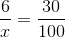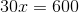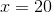## Example Questions

### Example Question #1 : Whole And Part

Mr. Owens spent $7.50 for a dinner buffet. The amount he paid accounted for 3/4 of the money in his wallet. How much money is left in his wallet for other expenses? Possible Answers:$2.50

$6.50$1.00

$4.00$10.00

$2.50 Explanation: If$7.50 is 3/4 of the total, 7.50/3 gives us what 1/4 of his total money would be. This equals \$2.50, the remaining unspent quarter.

### Example Question #2 : Whole And Part

A certain ball that is dropped will bounce back to 3/5 of the height it was initially dropped from.  If after the 2nd bounce the ball reaches  39.96 ft, what was the initial height the ball was dropped from?

135 ft

66 ft

100 ft

111 ft

150 ft

111 ft

Explanation:

We know the height of the initial bounce, so work backwards to find the initial height.  39.96/0.6 = 66.6 = height of ball after first bounce

66.6/0.6 = 111 ft

### Example Question #3 : Whole And Part

A pitcher of water is filled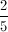of full.  An additional 27 ounces of water is added.  Now the pitcher of water is completely full.  How much water does the pitcher hold?

40

50

45

35

30

45

Explanation:

If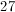ounces fills the pitcher, then it must equal the volume of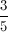of the pitcher.  Ifof a pitcher equals 27 ounces, then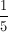of a pitcher equals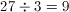ounces.  Since there arefifths in the pitcher, it must hold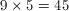ounces total.

### Example Question #11 : Whole And Part

If Mr. Jones’ math class has 8 boys and two-thirds of the class are girls, how many total students are in the class?Explanation:

If two-thirds of the class are girls, then one-third must be boys. Set up an equation comparing the number of boys to how much they represent in the entire class:

8 = (1/3) x, where x is the number in the entire class.

When we solve for x in the equation we get x = 24.

### Example Question #12 : Whole And Partis what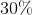of what?Explanation:

With the given information, we can set up a proportion.